## Introduction

Growth is a ubiquitous phenomenon in complex systems. Consider, for example, a modern infrastructure in a large metropolitan area. Due to the influx of population, the essential facilities such as the electrical power grids, the roads, water supply and all kinds of services need to grow accordingly. The issue of how to maintain the performance of the growing systems under certain constraints (e.g., quality of living) becomes critically important from the standpoint of sustainability. To develop a comprehensive theoretical framework to understand, at a quantitative level, the fundamental dynamics of sustainability in complex systems subject to continuous growth is a challenging and open problem at the present. In this paper, to shed light on how a complex network can maintain its function and how such a function may be lost during network growth, we focus on the dynamics of synchronization. In particular, if a small network is synchronizable, as it grows in size the synchronous state may collapse. The main purpose of the paper is to uncover and understand the dynamical features of synchronization collapse as the network grows. As will be explained, our main result is that the collapse is essentially a self-organizing dynamical process towards criticality with an algebraic scaling behavior.

From the beginning of modern network science, growth has been recognized and treated as an intrinsic property of complex networks1,2. For example, the pioneering model of scale free networks3 had growth as a fundamental ingredient to generate the algebraic degree distribution. The growth aspect of this model is, however, somewhat simplistic as it stipulates a monotonic increasing behavior in the network size, whereas the growth behavior in real world networks can be highly non-monotonic. For example, in technological networks such as the electric power grid, introducing a new node (e.g., a power station) will increase the load on the existing nodes in the network, which can trigger a cascade of failures when overload occurs4,5,6,7,8,9,10,11,12,13,14,15,16,17,18,19,20,21,22,23,24. In this case, the addition of a new node does not increase the network size but instead results in a network collapse5,24. A similar phenomenon was also observed in ecological networks, where the introduction of a new species may result in the extinction of many existing species25,26. In an economic crisis, the failure of one financial institute can result in failures of many others in a cascading manner21,27. To take into account the phenomenon of non-monotonic network growth so as to avoid network collapse, an earlier approach was to constrain the growth according to certain functional requirement such as the system stability with respect to certain performance, i.e., to impose the criterion that the system must be stable at all times25. It was revealed that network growth subject to a global stability constraint can lead to a non-monotonic network growth without collapse28. Constraint based on network synchronization was proposed29, where it was demonstrated that imposing synchronization stability can result in a highly selective and dynamic growth process29 in the sense that it often takes many time steps for a new node to be successfully “absorbed” into the existing network.

To be concrete, we study the growth of complex networks under the constraint of synchronization stability. Synchronization of coupled nonlinear oscillators has been an active area of research in nonlinear science30,31,32,33,34 and it is an important type of collective dynamics on complex networks35. Earlier studies focused on systems of regular coupling structures, e.g., lattices or globally coupled networks. The discovery of the small world36 and scale free3 network topologies in realistic systems generated a great deal of interest in studying the interplay between complex network structure and synchronization37,38,39,40,41,42,43,44,45,46,47,48,49,50,51. Since the structures of many realistic networks are not static but evolving with time52,53, synchronization in time-varying complex networks was also studied54,55,56 to reveal the dynamical interplay between the time-dependent network structure and synchronization39,57,58. We note that there was a line of works addressing the improvement of synchronization by evolving the network structure, such as link rewiring59,60, adjustment of coupling weights61,62, change the coupling scheme63,64, but in these works the network size is assumed to be fixed.

To investigate the growth of stability-constrained complex networks, a key issue is the different time scales involved in the dynamical evolution28,29,65. For network growth constrained by synchronization, in general there are two key time scales: one associated with the transient synchronization dynamics occurred in a static network, denoted as Ts and another characterizing the speed of network growth, e.g., the time interval between two successive nodal additions, Tg. The interplay between the two time scales can result in distinct network evolution dynamics. For example, for TsTg, the stability constraint would have little effect on the network evolution and, in an approximate sense, the network grows as if no constraint were imposed. However, for TsTg, the network remains synchronized at all times. In particular, since the stability is determined by the network structure, e.g., through the eigenvalues of the coupling matrix, the dynamics of network evolution is effectively decoupled from that of synchronization.

For Ts ≈ Tg, complicated network evolution dynamics can arise65, where the two types of dynamical processes, i.e., growth and synchronization, are entangled. Depending on the instant network structure and synchronization behavior, the addition of a new node may either increase or decrease the network size. For example, if the new node induces a desynchronization avalanche, a number of nodes will be removed if their synchronization errors exceed some threshold values, resulting in a sudden decrease of the network size and potentially a large scale collapse. In this paper, we focus on the regime of Ts ≈ Tg and introduce a tolerance threshold to determine if a node has become desynchronized. Specifically, after each transient period of evolution, we remove all nodes with synchronization error exceeding this threshold. During the course of evolution, the network can collapse at random times. Strikingly, we find that the size of the collapses follows an algebraic scaling law, indicating that the network growth dynamics under the synchronization constraint can be regarded as a process towards self-organized criticality (SOC).

## Results

### Model of network growth subject to synchronization constraint

We consider the standard scale-free growth model3 but impose a synchronization-based constraint for nodal removal. Specifically, starting from a small, synchronizable core of m0 coupled nonlinear oscillators (nodes), at each time step ng of network growth, we add a new node with random initial condition into the network. The new node is connected to m existing nodes according to the preferential attachment probability Πi = ki/∑jkj, where i, j = 1, 2, …, n are the nodal indices and ki is the degree of the ith node. We then monitor the system evolution for a fixed time period (Tg) and calculate the nodal synchronization error δri (to be defined below). Defining δrc as the tolerance threshold for nodal desynchronization, if all nodes in the network meet the condition δri < δrc, the network size will be increased by one. Otherwise, the nodes with δri > δrc will be removed from the network, together with the links attached to them. For convenience, we use the term “collapse” to describe the process of nodal removal and the number of removed nodes, Δn, is the collapse size.

For simplicity, we set the nodal dynamics to be identical and adopt the normalized coupling scheme66,67, where the dynamical evolution of the ith oscillator in the network is governed by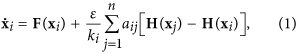with F and H representing, respectively, the dynamics of the isolated oscillator and the coupling function. The network structure is characterized by the adjacency matrix {aij}, where aij = 1 if oscillators i and j are directly connected and aij = 0 otherwise. The parameter ε > 0 is the uniform coupling strength. Note that the coupling strength from node j to node i, cij = (εaij)/ki, in general is different from that for the opposite direction, so the network is weighted and directed67. The class of models of linearly coupled nonlinear oscillators with variants are commonly used in the literature of network synchronization68. While Eq. (1) is for continuous-time dynamical systems, networks of coupled nonlinear maps can be formulated in a similar way.

To be concrete, we assume that the individual nodal dynamical process is described by the chaotic logistic map, x(t + 1) = F[x(t)] = 4x(t)[1 − x(t)] and choose H(x) = F(x) as the coupling function. The coupling strength is fixed at ε = 1. The initial network consists of m0 = 8 globally coupled nodes, which is synchronizable for the given coupling strength. For a fixed time interval Tg = 300, we introduce a new node (map) into the network with a randomly chosen initial condition in the interval (0, 1) by attaching it to the existing nodes according to the preferential attachment rule. The synchronization error is defined as δri = |xi − 〈x〉| ith 〈x〉 = ∑ixi/n being the network-averaged state, which is calculated at the end of each time interval Tg. We set the tolerance threshold to be δrc = 10−10 (somewhat arbitrarily). The growing process is terminated either if the network has completely collapsed (n ≈ 0) or when its size reaches a preset upper bound (e.g, 1000).

Figure 1 shows the network size n versus the time step ng. We see that, after an initial period of linear growth (ng ≤ 123), the network size is suddenly decreased from n = 128 to 103, signifying that a collapse event of size Δn = 25 has occurred after the addition of the 124th oscillator. After the collapse, the network begins to expand again. In the subsequent time evolution, collapse of different sizes occurs at random times, e.g., Δn = 22 at ng = 379 and Δn = 10 at ng = 418. For relatively small network size, when a collapse event occurs, the removed nodes account for only a small fraction of the nodes in the entire network (e.g., Δn/n < 10%), with growth followed immediately after the collapse. However, as the network size exceeds a critical value, say nmax = 400, this scenario of small-scale collapse followed by growth is changed dramatically. As shown in Fig. 1(a), for ng = 471, a catastrophic collapse event occurs, which removes over 75% of the nodes in the network (from 471 to 111). More strikingly, there is no growth after the event - the network continues to collapse. At the end of ng = 472, not a single node remains in the network, i.e., the network has collapsed completely.

To gain more insights into the dynamics of network collapse, we monitor the system evolution for the time period 123Tg < t < 124Tg, i.e., the response of the network dynamics to the addition of the 124th node. Figure 1(b) shows the time evolution of the averaged network synchronization error, 〈δr〉 = ∑iδri/n, where its value approaches zero rapid with time. A semi-logarithmic plot reveals an exponentially decreasing behavior for 〈δr〉 [inset of Fig. 1(b)], indicating that the network is able to restore synchronization for relatively large values of Tg. However, for Tg = 300, at the end of the time interval t = 124Tg, the synchronization errors of certain nodes exceed the threshold, leading to their removal from the network. The synchronization errors for three typical nodes are shown in Fig. 1(c). Examining the individual nodal synchronization errors δri, we find that, the “disturbance” triggered by the addition of a new node spreads quickly over the network, as shown in Fig. 1(d). After the disturbance reaches the maximal dynamical range at t ≈ 123Tg + 5 [Fig. 1(e)], it begins to shrink and, at the end of this time interval, there are still a few nodes with δr > δrc, as shown in Fig. 1(f). Based on their dynamical responses, the nodes can be roughly divided into three categories, as shown in Fig. 1(c). Specifically, for most nodes, as time increases δr first increases and then decreases, e.g., the 126th node. There are also nodes for which the values of δr decrease monotonically with time, e.g., the 125th node. Finally, there are a few nodes for which the values of δr remain about 0, e.g., the 129th node. We also observe that, sometimes, the new node, whose introduction into the network triggers a network collapse, in fact remains in the network.

### Statistical properties of collapse and self-organized criticality

In terms of practical significance, the following questions about network collapse are of interest: (1) what kind of nodes are more likely to be removed? (2) what is the size distribution of the collapse? (3) how frequent is the network collapsed? and (4) what are the effects of the tolerance threshold δrc and growing interval Tg on the collapse? In what follows, we address these questions numerically.

A simple way to identify the removed nodes is to examine their degrees. With the same parameters as in Fig. 1, we plot in Fig. 2(a) the normalized degree distribution, pdel(k), of the removed nodes collected from a large number of collapse events (except the catastrophic one that totally destroys the network). We see that the distribution contains approximately three distinct segments with different scaling behaviors. Specifically, for k [1, m], pdel(k) increases with k exponentially. For k [m, 40], pdel(k) decreases with k algebraically with the exponent γ ≈ −2.83. For k [40, 120], pdel(k) decreases with k exponentially. Since, in our model each new node has m = 8 links, it is somewhat surprising to see from Fig. 2(a) that some nodes have their degrees smaller than m. This phenomenon can be attributed to the node removal mechanism: when a node is removed, all links associated to it are also removed. Another phenomenon is that pdel(k) reaches its maximum at k = 8, which seems to contradict the previous result that nodes of large degrees are more stable with respect to synchronization than those of small degrees61,66,67,68.

Since pdel(k) is obtained from a large number of collapses, to uncover the interplay between nodal stability and degree, we need to take into account the degree distribution p(k) of the generated network. To find p(k), we use the largest network emerged in the growth process (the network formed immediately before the catastrophic collapse) and obtain the degree distribution for an ensemble of such networks. The results are also shown in Fig. 2(a). We see that the two distributions, pdel(k) and p(k), coincide with each other well, where p(k) also contains three distinct segments and reaches its maximum at k = m. The consistency between pdel(k) and p(k) suggests that the nodal stability is independent of the degree. Statistically, we thus expect that the small and large degree nodes to have equal probability to be removed.

Figure 2(b) shows the collapse size distribution, where the catastrophic network size nmax is not included. We see that, in the interval Δn [1, 100], the distribution follows an algebraic scaling: pcoln) ~ Δnγ, with γ ≈ −0.85. For Δn > 100, an exponential tail is observed. To test whether the exponential tail is a result of the finite size effect, we decrease the transient period to Tg = 200 and plot the distribution of the collapse size again. (As we will demonstrate later, as Tg is decreased, the maximum network size nmax will decrease monotonically.) Figure 2(b) indicates that, comparing with the case of Tg = 300, the regime of algebraic scaling is shifted toward the left for Tg = 200. Specifically, for Tg = 200, we have pcoln) ~ Δnγ in the interval Δn [1,50], where the fitted exponent is about −0.79.

The emergence of algebraic scaling in the size distribution of network collapse is interesting from the viewpoint of SOC that occurs in many real-world complex systems. For a dynamical system subject to continuous external perturbations, during its evolution towards SOC, it can appear stable for a long period of time before a catastrophic event occurs and the probability for the catastrophe can be markedly larger than intuitively expected (algebraic versus exponential scaling)69,70. In our case, there is a long time period of synchronization stability in spite of the small-size collapses, but catastrophic collapses that remove all or most of the nodes in the network can occur, albeit rarely. There are a variety of models for SOC, but the unique feature of our model is that it exploits network synchronization stability as a mechanism for catastrophic failures. Since synchronization is ubiquitous in natural and man-made complex systems, the finding of SOC in synchronization-stability-constrained network may have broad implications. For instance, synchronization is commonly regarded as the dynamical basis for normal functioning of the power grids71 and there is empirical evidence that the size of the blackouts follows roughly an algebraic distribution72.

We proceed to study the frequency of network collapse. Let Δn′ be the period of continuous network growth, i.e., the number of nodes successively added into the network between two adjacent collapses. The collapse frequency is f = 1/〈Δn′〉, where 〈Δn′〉 is the averaged period. For the same parameters in Fig. 1, we find f ≈ 1/21. That is, on average the network collapses every 21 new additions. Since the synchronization errors are evaluated at the end of each transient interval and nodes are removed according to a predefined tolerance threshold, we expect the collapse frequency to depend on the parameters Tg and δrc. This is apparent in Fig. 1(a), where the network growth under the parameters Tg = 300 and δrc = 10−9 is also shown. We see that, comparing with the case of δrc = 10−10, the catastrophic collapse is postponed. To assess the influence of Tg and δrc on f, we show in Fig. 3(a) f versus Tg for different values of δrc. It can be seen that, with the increase of Tg or δrc, f decreases monotonically.

For the process of network growth, two particularly relevant quantities are: (1) the critical network size n1 at which the first collapse occurs and (2) the maximum network size nmax beyond which a catastrophic collapse occurs. Similar to the collapse frequency, these two quantities depend on the parameters Tg and δrc. Figure 3(b) shows n1 (nmax) versus Tg for different values of δrc. We see that, as Tg or δrc is increased, n1 (nmax) increases monotonically. That is, by increasing Tg or δrc, one can postpone the first and the catastrophic network collapse but eventually it will occur.

### Physical theory of synchronization based network collapse

As the network synchronizability can be characterized by the stability distances dl,r (see Methods), we calculate the evolution of dl,r during the course of network growth, as shown in Fig. 4(a). In accordance with the process of network growth [Fig. 1], the time evolution of dl,r also consists of distinct regimes. Firstly, as ng increase from 1 to 123, dl,r approaches zero quickly. Secondly, in the interval ng (123, 470), dl,r remains about zero. A magnification of this interval reveals that, while dl,r tend to reach zero, the process is occasionally interrupted by some small increments. Checking the points at which dl,r increase suddenly [inset of Fig. 4(a)], we find that these points correspond to exactly the time instants of network collapses. For example, for ng = 379, dl increases from 0.032 to 0.041 [Fig. 4(a)], while at the same time there is a collapse event in which the network size changes from n = 344 to 322 [Fig. 1(a)]. Finally, at the critical instant ng = 472 where the catastrophic collapse occurs, dl and dr change suddenly to 0.21 and 0.22, respectively.

Figure 4 thus indicates that, for the entire process of network growth, the stability distances dl,r remain positive so that the network is synchronizable at all time. That is, even at the time when a collapse occurs, no node would be removed if the transient time Tg is sufficiently long. It may then be said that, with respect to the impact of the network synchronizability (as determined by the network structure), network collapse is equally influenced by the transient synchronization dynamics. Increasing Tg can thus effectively postpone the collapses as the network grows, a manifestation of which is a further decrease in dl,r at the collapses. Let dmin be the minimum of dl,r during the process of network growth. Figure 4(b) shows dmin versus Tg for different values of δrc. As anticipated, increasing the value of Tg or δrc results in a monotonic decrease in the value of dmin, which agrees with the results of direct simulations in Fig. 3(b) where a postponement of the catastrophic collapse is explicitly demonstrated.

The fact that dl,r become approximately zero prior to a catastrophic collapse implies that the network becomes marginally stable during the growing process, i.e., the oscillator trajectories deviate only slightly from the synchronous manifold. In this case, desynchronization is determined by the two extreme modes, σ2 and σn, as the corresponding transverse Lyapunov exponents Λ(σ2,n) are larger than those associated with other transverse modes73. This feature makes possible a theoretical analysis of the collapse phenomenon. In particular, assuming dl,r ≈ 0 and Λ(σ2) > Λ(σn) (so that the 2nd transverse mode is more unstable), we have that desynchronization is mainly determined by the 2nd mode, with ξ2(t) ~ exp[Λ(σ2)t]. Since Λ(σ2) ≈ 0, we have ξ2(t) ~ Λ(σ2)t . Transforming this mode back to the nodal space, we obtain δri = |e2,iξ2| ~ |e2,iΛ(σ2)t, where e2,i is the ith component of the eigenvector e2 associated with σ2. For the given network structure, the value of Λ(σ2) is fixed. We thus have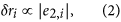which establishes a connection between the network structure and the oscillator stability. It is only necessary to calculate the eigenvector associated with the most unstable mode to identify the unstable oscillators,

Relation (2) can be verified numerically. As shown in the inset of Fig. 4(a), at the growing step ng = 379, the network contains n = 322 oscillators and the two extreme eigenvalues are (σ2n) = (0.538, 1.468). Since Λ(σ2) = −0.079 and Λ(σn) = −0.066, desynchronization is determined by the nth mode. Figure 5(a) shows the synchronization errors (measured at the end of the 379th growing step) δri versus the absolute eigenvector element |e2,i| for all the oscillators in the network, which is obtained from the network coupling matrix C. We see that δri increases with |en,i| linearly. The linear relationship is also observed when the 2nd transverse mode is more unstable. For example, at the growing step ng = 418, the network contains n = 350 oscillators and the two pertinent Lyapunov exponents are [Λ(σ2), Λ(σn)] = (−0.070, −0.081). The linear variation of δri with |e2,i| is also shown in Fig. 5(a).

Relation (2) can also be used to interpret the size distribution of the network collapses observed numerically [e.g., Fig. 2(b)]. Let δri(0) be the initial synchronization error of the ith oscillator induced by the newly added oscillator. After a transient phase of length Tg, the error becomes δri ≈ δri(0)|ej′,i|exp[Λ(σj)Tg], with j′ = 2 or n (depending on which mode is more unstable). As Λ(σj) is approximately zero, we have δri ≈ δri(0)|ej′,i|[1 + Λ(σj)Tg]. Setting δri = δrc, we get the critical element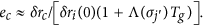Thus, whether the ith oscillator is removed solely depends on the element ej′,i. In particular, if |ej′,i| > ec, we have δri > δrc so that the oscillator will be removed; otherwise it will remain in the network. Assuming the oscillators have the same initial error δr(0), we can estimate the size of the network collapse simply by counting the number of elements satisfying the inequality |ej′,i| > ec. To verify this idea, we generate scale-free networks, calculate the eigenvector e2 and identify the largest element emax of e2. Choosing ec randomly from the range (0, emax) [since d(0) is dependent upon the (random) initial condition of the newly added oscillator], we truncate the eigenvector elements, where the number of truncated elements is the collapse size. We repeat this truncation procedure for a large number of statistical realizations and calculate the size distribution of the collapses. The result for a network of size n = 800 is shown in Fig. 5(b). We see that the size distribution calculated from the eigenvector analysis also follows an algebraic scaling: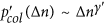, where the fitted exponent is γ′ ≈ −0.91. This is in good agreement with the one obtained from direct simulations [Fig. 2(b)], where the algebraic scaling exponent is γ ≈ −0.85 for the interval Δn [1, 100].

### Alternative models of network dynamics

To demonstrate the generality of the synchronization based network collapse phenomenon and its SOC characteristics, we simulate continuous time dynamics on networks that grow according to alternative rules other than the preferential attachment mechanism. In fact, in network modeling, the way by which a new node is added to the existing network can have a determining role in the network structure1. For example, in unconstrained growing networks, random attachment cannot lead to any scale free feature but results in an exponential degree distribution74. Since the network structure has a significant effect on synchronization, we expect the characteristics of network growth dynamics following random attachment to be different from those from the preferential attachment rule. Besides the network structure, our eigenvector analysis indicates that the synchronization behavior is also dependent upon the nodal dynamics and the coupling function. For example, for a different type of nodal dynamics, the MSF curve can be dramatically different, so is the stability parameter region75,76,77. We are led by these considerations to study continuous-time oscillator networks that grow according to the random attachment rule. For the coupled logistic map network with the random attachment rule, the dynamical and statistical properties of network growth are found to be similar to those with the preferential attachment mechanism. In particular, the size of the collapse event follows approximately an algebraic scaling, where the scaling exponent decreases with the value of the synchronization threshold.

We choose the chaotic Rössler oscillator78 described by (dx/dt, dy/dt, dz/dt) = (−y − z, x + 0.2y, 0.2 + xz − 9.0z). The oscillators at different nodes are coupled through the x variable with the coupling function H([x, y, z]T) = [0, y, 0]T. We define the synchronization error as δri = |xi − 〈x〉|. The coupling strength is fixed at ε = 0.35. The stable synchronization region from the MSF curve is open at the right side77, i.e., the transverse mode i is stable for σi > σl ≈ 0.157. Adopting the random attachment rule, we grow the network under the constraint of synchronization stability and find the phenomenon of network collapse to be robust. For example, Fig. 6(a) shows the critical network size n1 versus the transient time Tg for different values of the tolerance threshold δrc. We see that, while n1 increases monotonically with Tg and δrc, the rate is somewhat smaller than that associated with the preferential attachment rule [Fig. 3(b)], indicating that the random attachment rule tends to make network collapses more frequent. Figure 6(b) shows the algebraic distribution of the collapse size generated by parameters δrc = 1 × 10−5 and Tg = 400, which shows pcoln) ~ Δnγ for Δn [1, 50], with γ ≈ −0.58. These results suggest that the SOC characteristics of the network collapse phenomenon are robust, regardless of the details of the network growth mechanism and of the nodal dynamical processes.

For the randomly growing chaotic Rössler network, we find that the relationship between the synchronization error δri and the eigenvector element e2,i can still be described by (2) [inset in Fig. 6(b)]. However, when analyzing the algebraic size distribution using the eigenvectors, we note that the agreement between the theoretical predication and the direct simulation results is not as good as that for the preferential attachment growth rule. For example, by truncating the eigenvector e2 of a random network of n = 800 nodes, we obtainwith γ′ ≈ −0.94. The difference in the value of the algebraic scaling exponent can be attributed to the limited size of the network generated subject to the synchronization constraint as well as to the relatively short transient period (small values of Tg). In fact, in a computationally feasible implementation of the random growth model with continuous-time dynamics, the largest network generated has the size n ≈ 50, rendering somewhat severe the finite size effect. Nonetheless, in spite of the finite-size effect, the SOC features of the network collapse phenomenon are robust.

We note that the transient time for network synchronization, Ts, depends on the network size n through the eigenvalues of the network coupling matrix. The system size n, however, varies with time due to network growth. The transient time Ts also depends on the synchronization threshold δrc and the initial perturbation δro. In general, the value of Ts can be estimated numerically. For example, for the coupled logistic map network, during the network growth process the eigenvalue of the most unstable mode (σ2 or σn) is on the order of 10−2. Our theoretical analysis then gives Ts ≈ [ln(δr0/δrc)]/σ2,n. Since δr0 ≈ 1 and δrc ≈ 10−10, we have Ts ≈ 300, which is the value used in Figs 1, 2, 3, 4, 5. The transient time for the coupled Rössler oscillator network can be estimated similarly (Fig. 6).

In our study, Ts was introduced mainly for the purpose of distinguishing growing networks from static (Ts = 0) and non-constrained (Ts → ∞) networks. The phenomena observed, i.e., network collapse and self-organized criticality (SOC), are not sensitive to the value of Ts. For instance, network growth dynamics similar to that in Fig. 1(a) can be observed for Ts = 50 and 500. It is worth mentioning that, despite progress made in recent years, to predict precisely the transient time of synchronization for complex networked dynamical systems remains to be a challenging problem.

## Discussions

Growth, or expansion, is a fundamental feature of complex networks in nature, society and technological systems. Growth, however, is often subject to constraints. Traditional models of complex networks contain certain growth mechanism, such as one based on the preferential attachment rule3, but impose no constraint. Apparently, when growth is constrained, typically the network cannot expand indefinitely, nor can its size be a monotonous function of time. As a result, during the growth process there must be times when the network size is reduced (collapse). But are there generic features of the collapse events? For example, statistically what is the distribution of the collapse size and are there universal characteristics in the distribution?

This paper addresses these intriguing questions using synchronization as a concrete type of constraint. In particular, taking into account the effects of desynchronization tolerance and synchronization speed, we propose and investigate growing complex networks subject to the constraint of synchronization stability. We find that, as new nodes are continuously added into the network, it can self-organize itself into a critical state where the addition of a single node can trigger a large scale collapse. Statistical analysis of the characteristics of the collapse events such as the degree distribution of the collapsed nodes, the collapse frequency and the collapse size distribution, indicates that constraint induced network collapse can be viewed as an evolutionary process towards self-organized criticality. The SOC feature is especially pronounced as the collapse size follows an algebraic scaling law. We develop an eigenvector analysis to understand the origin of the network collapse phenomenon and the associated scaling behaviors.

In a modern society, cities and infrastructures continue to expand. In social media, various groups (social networks) keep growing. When constraints are imposed, e.g., manifested as governmental policies or online security rules, how would the underlying network respond? Can constraints lead to large scale, catastrophic collapse of the entire network? These are difficult but highly pertinent questions. Our findings provide some hints about the dynamical features of the network collapse phenomenon, but much further efforts are needed in this direction of complex systems research.

## Methods

### Eigenvector analysis of network synchronizability

Say at step n′ of the growth, the network contains n − 1 synchronized oscillators and a new oscillator of random initial condition is introduced. Due to the new oscillator, the trajectories of the existing oscillators leave, at least temporarily, the synchronous manifold xs. Let δxi = xi − xs be the distance of the ith oscillator from the manifold, which is the synchronization error. The evolution of δxi is governed by the following variational equation: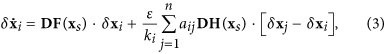where DF(xs) and DH(xs) are the Jacobian matrices of the local dynamics and the coupling function evaluated on xs, respectively. Equation (3) is obtained by linearizing Eq. (1) about the synchronous manifold xs, which characterizes its local stability75. To keep the expanded network synchronizable, a necessary condition is that all the synchronization errors, {δxi} approach zero exponentially with time. Projecting δxi into the eigenspace spanned by the eigenvector ei of the network coupling matrix C = εaij/ki, we can diagonalize the n coupled variational equations into n decoupled modes in the blocked form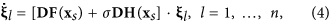where ξl is the lth mode transverse to the synchronous manifold xs and 0 = σ1 > σ2 … σn are the eigenvalues of the coupling matrix C. Among the n modes, the one associated with σ = 0 represents the motion within the synchronous manifold. The network is synchronizable only when all the transverse modes (ξj, j = 2, …, n) are stable, i.e., the largest Lyapunov exponent among these modes should be negative: Λ(σ) < 0. For typical nonlinear oscillators and smooth coupling functions, previous works75,76,77 showed that Λ(σ) can be negative within a bounded region in the parameter space of σ, i.e., Λ(σ) < 0 for σ  (σl, σr). Thus, the necessary condition to make the synchronous state stable is σl < σj < σr for all the transverse modes (j = 2, …, n). For the chaotic logistic map used in our numerical simulations, we have σl = 0.5 and σr = 1.5.

The eigenvalue analysis, also known as the master stability function (MSF) analysis, is standard in synchronization analysis75,76. It not only indicates whether a network is synchronizable, but also quantifies the degree of synchronization stability as well as the synchronization speed in certain situations79,80,81. Specifically, by examining the Lyapunov exponents associated with the two extreme modes, Λ(σ2) and Λ(σn), one can predict whether the network is synchronizable and how stable (unstable) the synchronous state is. In general, the smaller Λ(σ2) and Λ(σn) are, the more stable the synchronous state is75,76,77. Because of the relation Λ(σ2,n)  σ2,n, near the critical points σl and σr, the network synchronizability can be characterized by the stability distances dl = σ2 − σl and dr = σr − σn. For a synchronizable network, we have dl,r > 0. Moreover, the larger dl and dr are, the more stable the synchronous state will be. Otherwise, if one of the distances is negative, the synchronous state will be unstable. In the asynchronous case, the smaller dl and dr are, the more unstable the synchronous state will be.

### Simulations

For the coupled logistic map networks, the dynamics are simulated by iterating the maps in parallel according to Eq. (1). For the coupled Rössler oscillator networks, the 4th-order Runge-Kutta method was used to obtain the dynamical evolution of the system with the time step δt = 10−3.